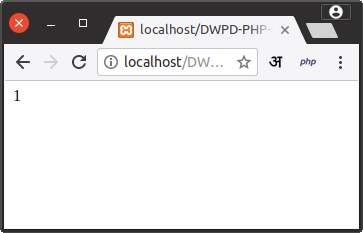Lab # 2
Basics of PHP

2. Write a PHP script to demonstrate arithmetic operators, comparison operation, and logical operation.

Lab-2-2-operators.php
```<?php
\$a="&";
\$b=(\$a==="&");
\$c=(\$b!=(\$a!="&"));
\$d=\$b*\$c;
echo \$d;
?>
```

I know this example is weird ;) but It's your challenge please explore yourself.

OutputLogical Operators example by Practical Server

Happy Coding :)